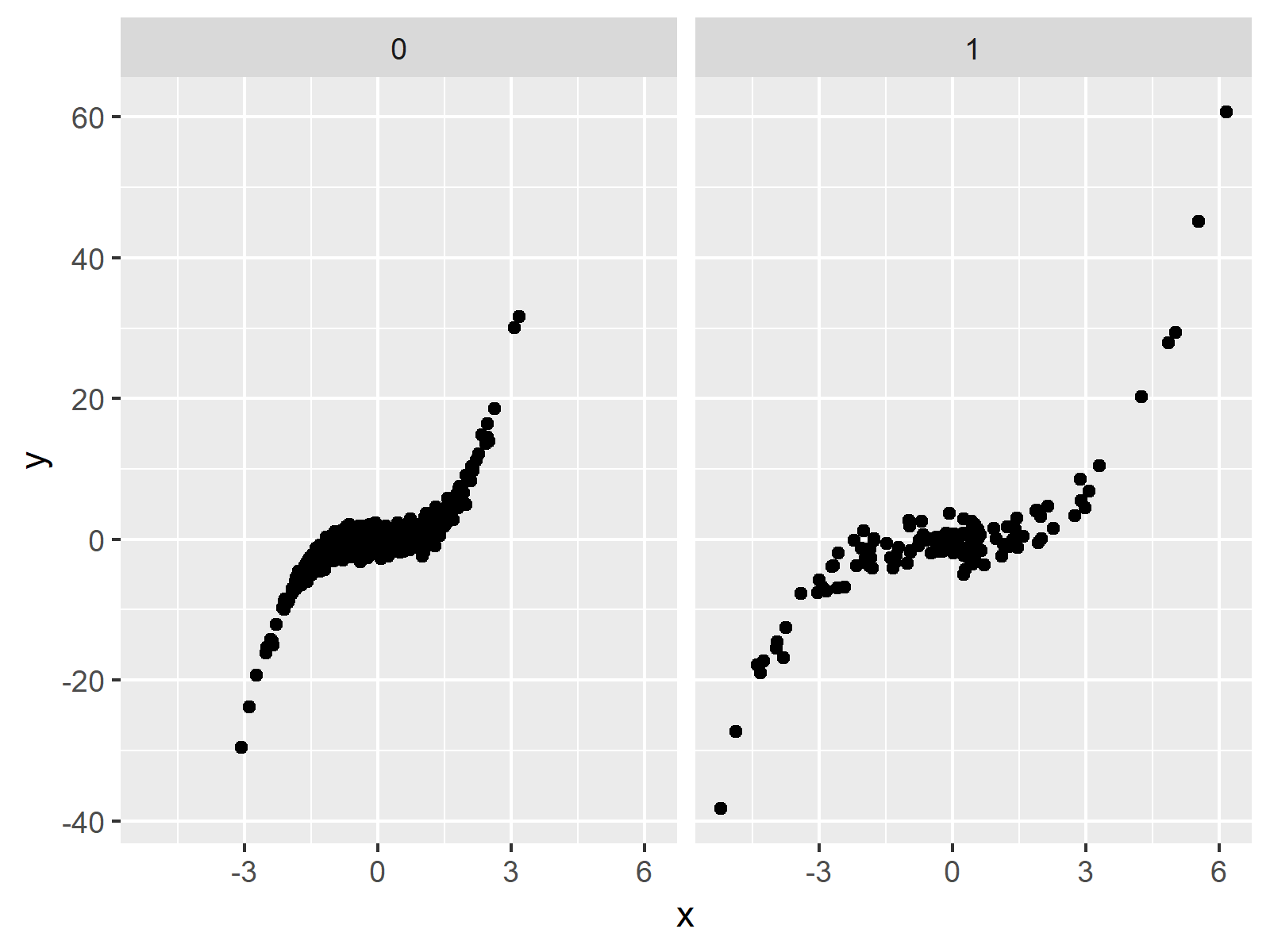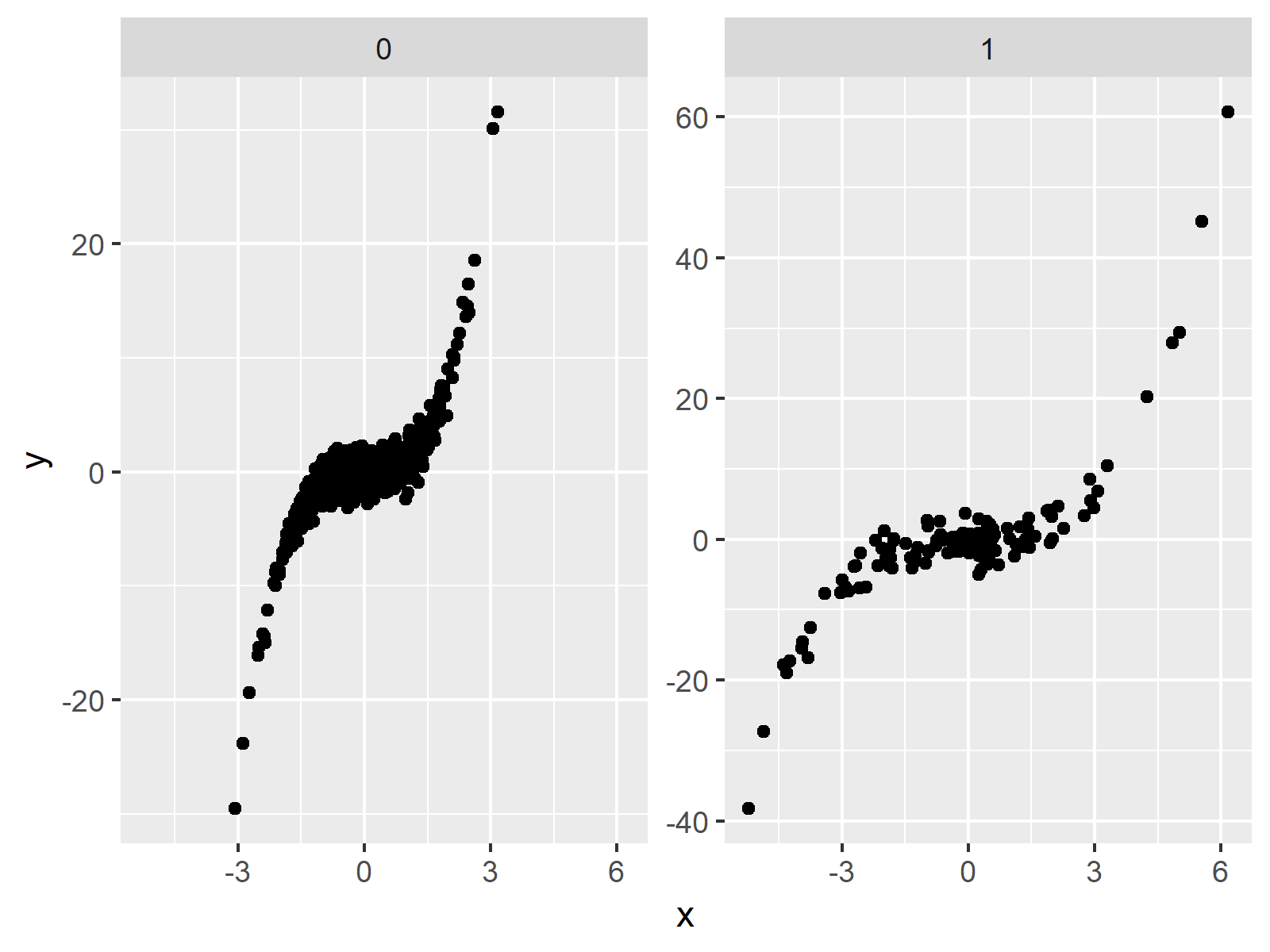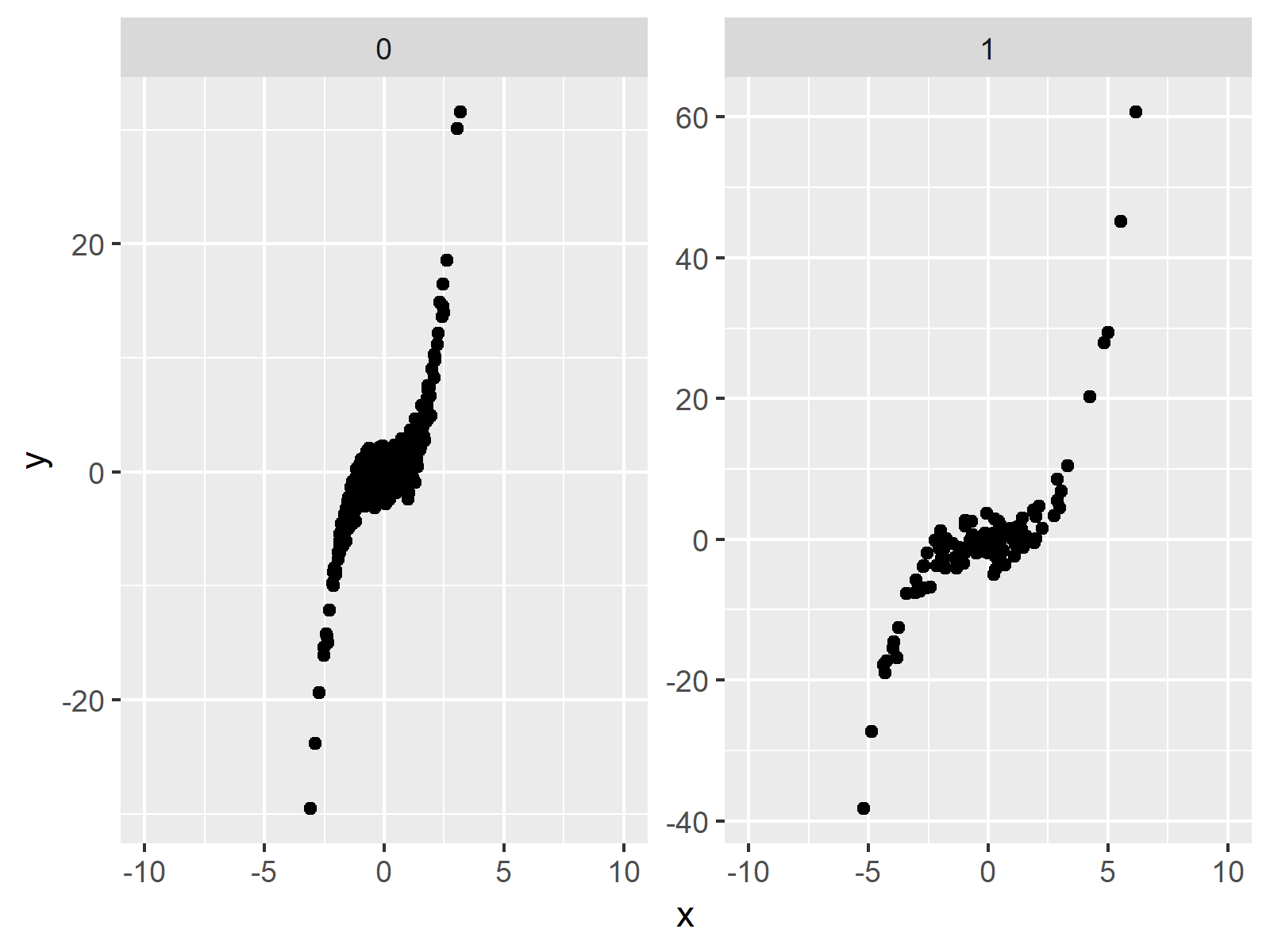# Set Axis Limits of ggplot2 Facet Plot in R (4 Examples) | Using facet_wrap & scales

This page shows how to manipulate the axis limits of a ggplot2 facet plot in R programming.

Sound good? Here’s how to do it!

## Creation of Exemplifying Data

First, we need to create some example data that we can draw as facet plots later on:

```set.seed(374658)                             # Create example data
x <- rnorm(1000)
y <- rnorm(1000) + x^3
group <- rbinom(1000, 1, 0.1)
x[group == 1] <- x[group == 1] * 2
y[group == 1] <- y[group == 1] * 2
data <- data.frame(x, y, group)
head(data)                                   # Print first lines of data
#             x          y group
# 1  0.27298625  1.0002430     0
# 2 -2.15253919 -9.7464363     0
# 3  0.01161435 -1.8907440     1
# 4 -0.65622032  0.7712863     0
# 5 -0.44922753  0.6075897     0
# 6  0.09518892  0.3644900     0```

As you can see based on the output of the RStudio console, our example data consists of three columns. It contains two numeric columns x and y as well as a group indicator.

If we want to draw data with the ggplot2 package, we need to install and load the package to R:

```install.packages("ggplot2")                  # Install & load ggplot2
library("ggplot2")```

Now, we can create a facet plot with default specifications as shown in the R code below:

```ggp <- ggplot(data, aes(x, y)) +             # Default plot with facet_wrap
geom_point() +
facet_wrap(~ group)
ggp                                          # Draw plot in RStudio```Figure 1: Facet Plot with Default Scales.

Figure 1 shows the output of the previous R syntax: A facet plot consisting of two ggplot2 scatterplots.

In the following examples, I’ll explain how to manipulate the axis scales of the panels of our plot. Keep on reading!

## Example 1: Create Facet Plot with Free Scales

As you have seen in the previous plot, by default the x-axis and the y-axis of our panels are set to be the same. Example 1 illustrates how to disentangle the scales of both plots, so that each scale fits the values shown in each panel:

```ggp +                                        # Draw plot with free scales
facet_wrap(~ group, scales = "free")```Figure 2: Facet Plot with Free Scales.

In Figure 2 you can see that our new facet graph shows panels with different scales on the x-axis as well as on the y-axis.

## Example 2: Create Facet Plot with Free X-Axis

In Example 2, you’ll learn how to keep the y-axis the same for both panels, while the x-axis is free:

```ggp +                                        # Draw plot with free x-axis
facet_wrap(~ group, scales = "free_x")```Figure 3: Facet Plot with Free X-Axis.

## Example 3: Create Facet Plot with Free Y-Axis

In Example 3, we keep the x-axis at a fixed size and disentangle the y-axes (i.e. the opposite as in Example 2):

```ggp +                                        # Draw plot with free y-axis
facet_wrap(~ group, scales = "free_y")```Figure 4: Facet Plot with Free Y-Axis.

## Example 4: Create Facet Plot with Individual Axes

In the previous examples, we used the predefined scales that are showing all values of each panel with the least possible empty space. However, you may also choose the axis limits manually by using the coord_cartesian function and the xlim (or ylim) argument.

The following R code creates a facet graphic with free y-axis and an individually specified x-axis:

```ggp +                                        # Free y-axis & manual x-axis
facet_wrap(~ group, scales = "free_y") +
coord_cartesian(xlim = c(- 10, 10))```Figure 5: Facet Plot with Manual X-Axis.

## Video, Further Resources & Summary

If you need further info on the R programming syntax of this article, you could watch the following video of my YouTube channel. I’m explaining the R syntax of this article in the video.

Please accept YouTube cookies to play this video. By accepting you will be accessing content from YouTube, a service provided by an external third party.If you accept this notice, your choice will be saved and the page will refresh.

Furthermore, you may want to have a look at the related tutorials on this website:

To summarize: You learned in this article how to modify and change the axis limits of different panels in a ggplot2 facet plot in the R programming language. Please tell me about it in the comments, in case you have additional comments or questions.

Subscribe to the Statistics Globe Newsletter

•Cara Wilson
March 30, 2021 7:37 pm

Very nice explanation! How would you specify the limits of all three axes? For example if you wanted to set the y limit on one plot to (-10,20) and the set the other one to (-10,10)?

•April 7, 2022 5:18 pm

I found this tutorial helpful. Thank you. Also, thanks for the referral regarding the question about different y-axis scales.

•April 8, 2022 5:52 am

Thank you very much for the kind feedback! I’m happy to hear that you find my tutorials helpful!

Regards,
Joachim

•March 25, 2023 10:19 am

I found what I needed from this post, thank you very much.
Regars
•March 27, 2023 10:23 am